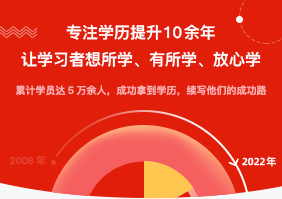2022
01 / 19
16 : 58

# 2021年成人高考高起点《理数》模拟试题及答案一

【导语】中国教育在线成人高考频道根据《全国各类成人高等学校招生复习考试大纲》收集整理了2021年成人高考高起点《理数》重点考点以供考生参考，具体如下：

2021年成人高考高起点《理数》模拟试题及答案一

1.设集合A={x|x=1},B={x|x3=1},则ANB=()

A.0

B.{1}

c.{-1}

D.{-1,1)

A={x|x3=1}={-11},B={x|x=1}={1},A0B={1}.

2、函数()-1]=lg的定义域是()

A.(0,+x0)

B.(-xc,0)

c.(0,1)

D.(1,+xc)

3.下列函数的图像与y=f(x)的图像关于原点对称的是()

A.y=-f(x)

B.y=f(-x)

Cy=-f(-x)

D.y=|f(x)

4.下列通数中，在区间(0,+xc)上是增函数的是()

A.y=-x

B.y=x-2

c.y=()

D.y=log:

B项中y=2x,当x>0时，y>0,故y=x-2在(0,+x)为增函数。

5.直线3x+y-2=0经过()

A.x一I

B.第一、二、四象限

C.第一、二、三象限

D.第二、三、四象限#### 版权声明

【声明】本文内容系湖南中医药类成考网编辑，未经授权不得转载，如需转载请注明出处。

阅读

阅读

阅读

阅读

阅读

## 网上报名. 马上进行成人高考报名 .

2022年成考报名预计

05-20

05-20

05-20

05-20

05-20